# An introduction to Q# — Microsoft’s language for quantum computing

Written by Ankit Sharma.

Photo by McDobbie Hu on Unsplash

In this article, I’ll introduce you to Q# — the new programming language from Microsoft for quantum computing. We will cover Q# data types, expressions, and statements with the help of code snippets.

#### Prerequisites

For an overview of quantum computing, please visit my earlier article: An Introduction To Quantum Computing. There, I also describe how to install Quantum Development Kit (QDK) in Visual Studio 2017.

### What is Q#?

According to Microsoft:

Q# is a scalable, multi-paradigm, domain-specific programming language for quantum computing.

So, what do these terms actually mean? Let us dive into the details.

• Scalable
Q# allows us to write code that can be executed on machines of varying computing abilities. We can use it to simulate a few Qubits on our local machine, or even thousands of Qubits for an enterprise level application.
Q# is a multi-paradigm programming language. It supports both functional and imperative programming styles. If you are new to programming paradigms, I suggest you refer here.
• Domain-specific
Q# is a programming language for quantum computing. It is to be used for writing algorithms and code snippets that are executed on quantum processors.

### Getting started with Q# development

This article will assume you have already installed QDK for Visual Studio 2017. If not, then you can check my earlier article for instructions.

After you have successfully installed QDK, we need to verify if Visual Studio 2017 has all the required dependencies installed for Q# development. For this, we will clone and execute the quantum sample programs from GitHub provided by Microsoft.

Open VS 2017 and navigate to Team >> Manage Connections.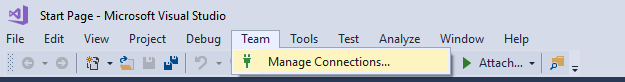Select Clone under Local Git Repositories and enter the URL: https://github.com/Microsoft/Quantum.git and click “Clone”.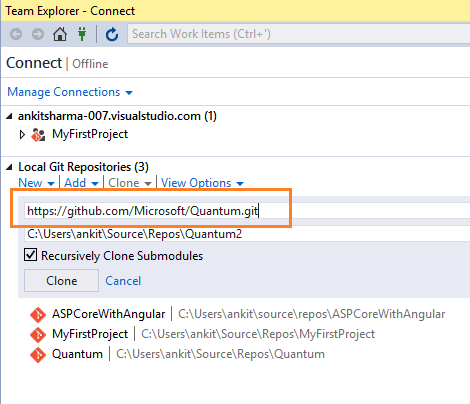The repository will be cloned on your local computer and Visual Studio will switch to the Solution Explorer. It will display all the cloned libraries and samples.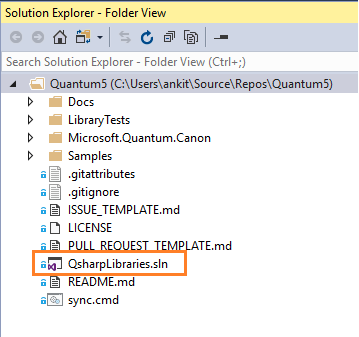Now, open QsharpLibraries.sln solution.

If you are prompted with the “Install Missing Features popup box, click “Install” to allow the installation of the necessary features. This will download and install F# and other tools used by some of the samples. Make sure that you are connected to the internet.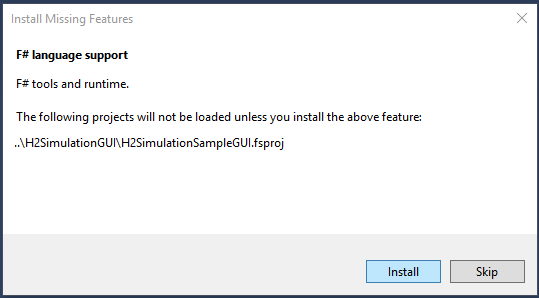To execute a sample program, right-click on the TeleportationSample project in “Samples > 0.Introduction folder” of QsharpLibraries solution, and then click on “Set as Startup Project” and press F5.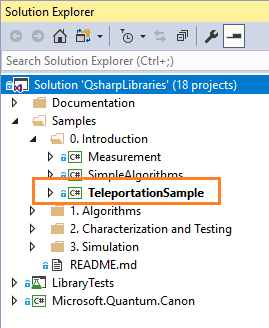If you can see an output screen similar to the one shown below, then congratulations, your VS 2017 is ready for Q# development.

Note that your output screen may vary because the data being teleported is random. But it should send 8 rounds of data, with all being successfully teleported.

### Q# Type model

Let us understand what are the various type models provided by Q#:

#### Primitive Type

• Int: — It represents the 64 -bit signed integer. Notice the upper case ‘I’. This is in contrast to int in C# with lower case ‘i’.
• Double: — It represents double-precision floating point number. This also has a upper case ‘D’ in contrast to double in C#.
• Bool: — It represents the Boolean type and can take two values — true or false.
• Qubit: — This represents the Quantum bit. Qubit is the fundamental unit of processing information in quantum computers, similar to a bit in classical computers.
• Pauli: — This type is used to denote the base operation for rotations and to specify the basis of a measurement.
• Result: — This represents the result of a measurement. This can take two possible values Zero or One
• Range: — This represents a sequence of integers.
• String: — It represents a sequence of Unicode characters.

#### Array Type

We can create an array type of any valid Q# primitive type. Q# does not support rectangular multi-dimensional arrays. Instead, it supports only jagged arrays.

Int[], Qubit[][]

By default, all variables in Q# are immutable. Their values cannot be changed after they are bound. So, to create an array whose values can be set, we will use the mutable keyword:

mutable myArr = new Int ;

This will create an integer array myArr of size 5. The elements of a new array are initialized to a type-dependent default value. In this case it will be 0, the default value for an integer type.

Arrays passed as arguments are immutable. All arrays in Q# are zero-based. That is, the first element of an array arr is always arr.

#### Tuple Type

The tuple type represents a tuple of values of any given primitive type. It is represented as (T1, T2, T3,…) where T1, T2, T3 are primitive types. The Q# tuple is immutable. We cannot change the contents of the tuple once it has been created.

A tuple expression can contain values of multiple primitive types. So, a tuple of type (Int, Double, Result) is a valid tuple.

We can create a tuple with single element also, like (2). This is known as a singleton tuple, and it is considered equal to the value of the enclosed type. This property is called singleton tuple equivalence.

For example, (2) is a singleton tuple of type Int, but it is considered equivalent to an integer 2.

We can create a user defined type of any primitive type. We can also create an array of user defined types or can also include it in a tuple. User defined types cannot have cyclic dependency on each other. So, it is not possible to create a recursive type structure.

A user-defined type is a subtype of the base type. This means it can be used anywhere a value of the base type is expected.

#### Operation Type

A Q# operation is a callable routine, which contains Q# code to carry out a quantum operation. An operation is the basic unit of quantum execution in Q#. The operation can only take single value as input in the form of a tuple. It returns a single value as output, specified after a colon, and may be a tuple.

An operation has a body section which contains the implementation of the operation. It can also have adjoint, controlled, and controlled adjoint sections. These are used to specify specific variants of appropriate operations. The arguments to an operation are specified as a tuple, within parentheses. The return type of the operation is specified after the colon.

Refer to a sample operation below:

`operation AddInteger(a: Int, b: Int): Int {      body {          mutable c = 0;          set c = a + b;          return (c);      }  }`

Here, we have an operation AddInteger which takes a tuple (Int, Int) as input. It returns an output of type Int after performing addition operations on input integers.

#### Function Type

A Q# Function is classical subroutine used within a Quantum algorithm and can only contain classical code (but no quantum operations). Similar to Q# operations, a function will also take a single value as input and returns a single value as output. Both of them can be a tuple. Functions cannot allocate qubits or call operations.

Let’s look at a sample function.

`function ProductNumber(a: Double, b: Double): Double {      mutable c = 0.0;      set c = a * b;      return (c);  }`

Here, we have defined a function ProductNumber, which takes a tuple (Double, Double) as input and returns an output of type Double after performing the product of input values. Also, notice that a function does not have a body section, as in the case of an operation.

### Expressions in Q#

Let’s take a look at various expressions provided in Q#.

#### Numeric Expressions

There are two types of numeric expressions provided by Q#:

• Integer numbers: these are represented by Int
• Floating point numbers: represented by Double

To represent a hexadecimal integer, we use the “0x” prefix.

We can also perform binary operations on numeric expressions to form a new numeric expression. The type of the new expression will be Double if both input expressions are floating point numbers, or will be an Int if both are integers.

Apart from binary operations, the numeric expressions also support modulus, power, bitwise AND, bitwise OR, bitwise XOR, and bitwise complement operations.

#### Qubit Expressions

Qubit expressions are the symbols that are bound to qubit values or the elements of a qubit array. Q# does not provide any support for qubit literals.

#### Pauli Expressions

As we have discussed earlier, the primitive type Pauli can take four possible values: PauliI, PauliX, PauliY and PauliZ. These all are valid Pauli expressions. We can also create an array of Pauli types, and the array elements are considered as valid Pauli expressions.

The two possible result values Zero and One are valid Result expressions. One important point to note is that One is not the same as integer 1, and Zero is not same as integer 0. Also, there is no direct conversion between them.

This is in contrast to C# where, boolean true is considered the same as integer 1 and boolean false is considered the same as integer 0.

#### Range Expressions

A range expression is represented as start..step..stop where start, step, stop are all integers. The range expression can take values as start, start+step, start+step+step and so on until stop is passed.

If only start and stop are mentioned in a range expression, then it will take the value of the step as set to 1 implicitly.

Let’s understand this with the help of an example:

• 1..3 — this indicates the range 1,2,3. This gives 1, 1+1, 1+1+1
• 1..2..6 indicates the range 1,3,5, or 1, 1+2,1+2+2
• 8..-2..3 indicates the range 8,6,4 or 8, 8+(-2), 8+(-2)+(-2)

#### Array Expressions

In Q# an array can be represented as a set of element expressions separated by semicolons and enclosed within square brackets. Similar to C#, all elements of an array in Q# should have the same type.

So, [1;2;3] is a valid array, but [1;2.5;Zero] is an invalid array.

We can also use the ‘+’ operator to concatenate two arrays of the same type.

So, [2;4;6] + [8;10;12] will give [2;4;6;8;10;12] as output.

To find the length of an array, we use the Length built-in function.

As an example, if myArr is an integer array having 5 elements, then Length(myArr) will return 5 as the output.

### Q# Statements

Symbols in Q# can be mutable or immutable.

An immutable symbol cannot be changed after it has been bound. We use the let keyword to define and bind an immutable symbol.

let i=8;

This will bind the symbol i as an integer with value 8. If we try to reset the value of an immutable expression, we will get a compile time error.

Hence set i=10; will give an error in this case.

A mutable symbol value can be changed after it has been bound. We use the mutable keyword to define and bind a mutable symbol.

mutable i=8;

This will bind the symbol i as an integer with value 8.

To change the value of a mutable symbol, we use the set keyword:

set i=10;

This will update the value of variable i to 10

#### for-loops

Q# allows a for-loop to iterate over an integer range. The for statement consists of the keyword for, followed by an identifier, the keyword in, a Range expression, and a statement block.

A range is specified by the first and last integers in the range, for example: 1..5 represents the range 1, 2, 3, 4, and 5. If a step other than +1 is needed, then three integers with .. between them are used.

So, 1..2..10 is the range 1, 3, 5, 7, and 9. The range is inclusive at both ends.

`for(num in 1..2..10)  {     //Do something  }`

As the name suggests, this loop will repeat until successful operation occurs. This loop is based on the quantum “repeat until success” pattern. It consists of the keyword repeat and its statement block, the keyword until, a Boolean expression, the keyword fixup, and its statement block .

The statement inside the repeat block is executed, and then the boolean condition is evaluated. If the boolean condition evaluates to true, then the loop terminates. Otherwise, the fixup block is executed and the loop repeats once again.

The fixup block is always required — even if there is no fixup to be done — in which case it will be empty.

`repeat {      //do something  }  until boolean condition  fixup {      // do something  }`

Q# supports if statements for conditional execution, similar to C#. The if statement consists of the keyword if, followed by a Boolean expression and the statement block. An if block may have an optional else block, which is represented by the keyword else.

`if (num % 2 == 0) {      return true;  } else {      return false;  }`

A conditional statement can consist of a series of if-elseif-else chains. The else-if clause is represented by the keyword elif.

`if (num == 1) {      //do something  }  elif(num == 2) {      //do something  }  else {      //do something  }`

### Conclusion

In this article, we have learned the basics of the Q# programming language. We also installed QDK and verified the Q# execution environment with Visual Studio 2017. Please post your valuable feedback in the comments section and stay tuned for more on Quantum Computing.

You can always refer to my previous articles here.